## 🍋常用的分类问题衡量标准

• 准确度（Accuracy）
准确度是最简单的性能衡量标准之一。它表示模型正确分类的样本数与总样本数之比。
适用情况：准确度适用于各个类别数量差距不大的情况，其中每个类别的重要性相似。

• 精确率（Precision）
精确率是指模型正确预测正类别样本的比例，它是真正例（True Positives, TP）与真正例与假正例之和（TP + False Positives, FP）的比值。
适用情况：当假正例的成本很高时，精确率是一个重要的度量标准，例如，医学诊断中。

• 召回率（Recall）
召回率是指模型正确预测正类别样本的比例，它是真正例（TP）与真正例与假负例（False Negatives, FN）之和的比值。
适用情况：当假负例的成本很高时，召回率是一个关键的性能度量，例如，安全检测中。

• F1分数（F1 Score）
F1分数是精确率和召回率的调和平均值，它可以平衡精确率和召回率之间的权衡关系。
适用情况：在需要同时考虑精确率和召回率的情况下，F1分数是一个有用的度量标准。

• ROC曲线和AUC（Receiver Operating Characteristic Curve and Area Under Curve）：
ROC曲线是一个用于可视化分类器性能的工具，而AUC是ROC曲线下的面积，用于量化分类器的性能。
适用情况：ROC曲线和AUC通常用于二进制分类问题，特别是在不同阈值下比较模型性能时。

## 🍋混淆矩阵-精确率-召回率

from sklearn.metrics import precision_score,recall_score,confusion_matrix
import numpy as np
from sklearn.model_selection import train_test_split


digits  =load_digits()
y = digits.target.copy()
X = digits.data
y [digits.target==8]=1
y [digits.target!=8]=0


y采取copy是因为，后面会报错，所以还是不要在原数据进行修改

X_train,X_test,y_train,y_test = train_test_split(X,y,random_state=666)
log_reg = LogisticRegression(max_iter=1000)
log_reg.fit(X_train,y_train)


max_iter参数用于指定模型训练的最大迭代次数，这里设置为1000，以确保模型在训练期间能够收敛到一个合适的解。

log_reg.score(X_test,y_test)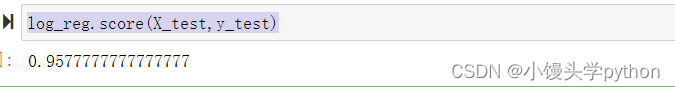confusion_matrix(y_test,log_reg.predict(X_test))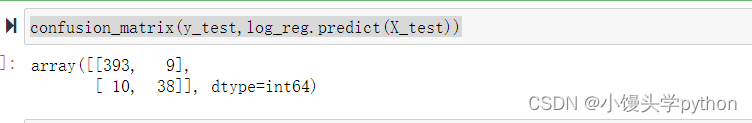precision_score(y_test,log_reg.predict(X_test))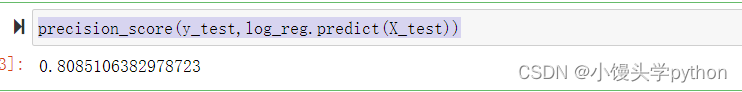recall_score(y_test,log_reg.predict(X_test))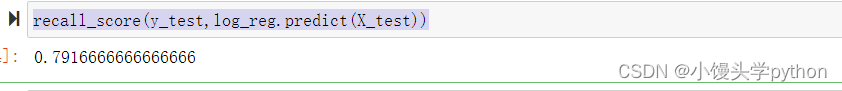from sklearn.metrics import f1_score
f1_score(y_test,log_reg.predict(X_test))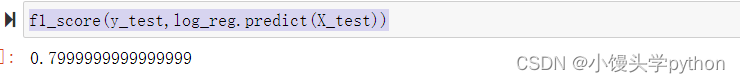## 🍋PR曲线和ROC曲线

### 🍋PR曲线

PR曲线是评估二分类模型性能的一种重要工具。它关注的是模型在正类别上的性能，特别是在识别正类别时的准确性和召回率。

Precision=TPTP+FPPrecision=TP+FPTP​

Recall=TPTP+FNRecall=TP+FNTP​

PR曲线以不同的阈值作为横轴，Precision和Recall作为纵轴，通过绘制不同阈值下的Precision-Recall点来可视化模型性能。一条PR曲线越靠近左上角，模型性能越好。

log_reg.decision_function(X_test)[:5]


from sklearn.metrics import precision_recall_curve


pre,recall,thresholds = precision_recall_curve(y_test,log_reg.decision_function(X_test))


import matplotlib.pyplot as plt
plt.plot(pre,recall)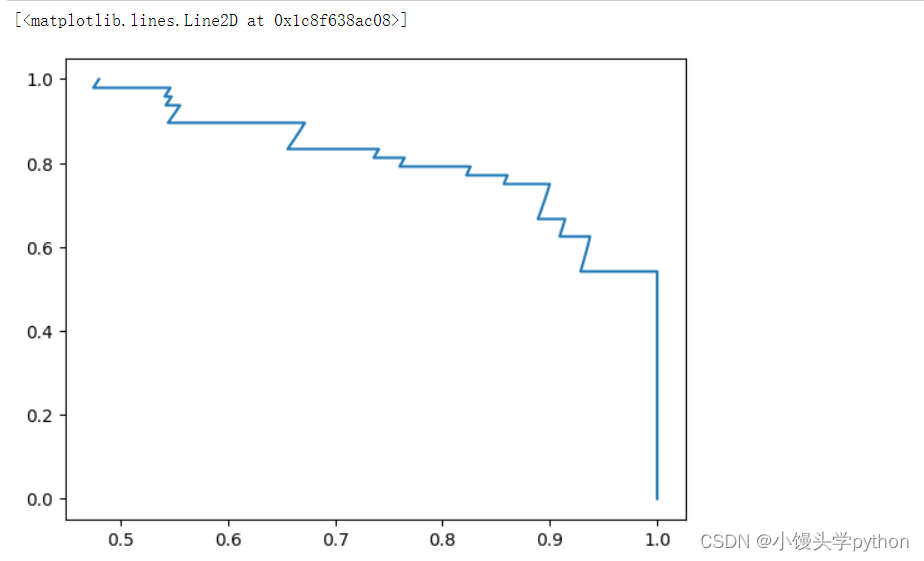### 🍋ROC曲线

ROC曲线是另一种用于评估分类模型性能的工具，它关注的是模型的真正例率（True Positive Rate）和假正例率（False Positive Rate）。

FPR=FPFP+TNFPR=FP+TNFP​

ROC曲线以不同的阈值作为横轴，True Positive Rate作为纵轴，通过绘制不同阈值下的True Positive Rate和False Positive Rate点来可视化模型性能。一条ROC曲线越靠近左上角，模型性能越好。

from sklearn.metrics import roc_curve,roc_auc_score
fpr,tpr,thresholds =  roc_curve(y_test,log_reg.decision_function(X_test))
plt.plot(fpr,tpr)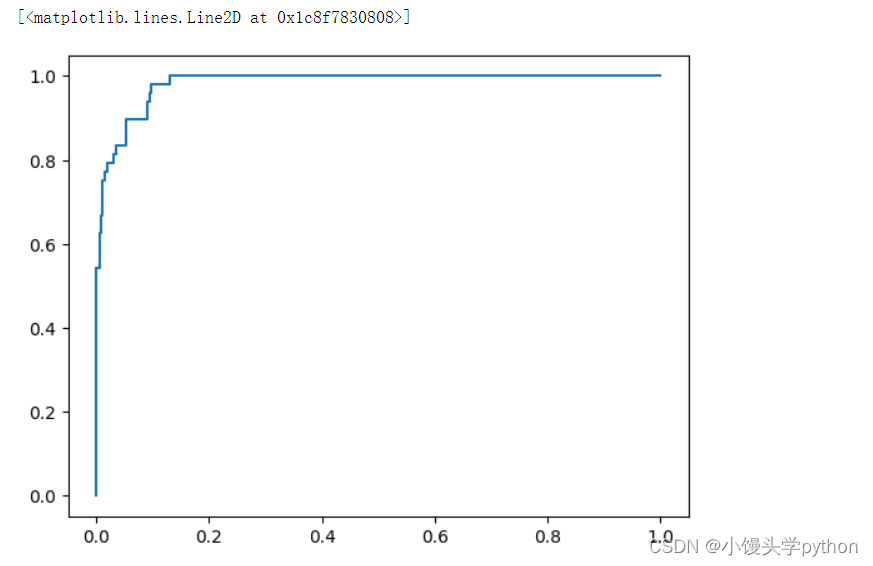roc_auc_score(y_test,log_reg.decision_function(X_test))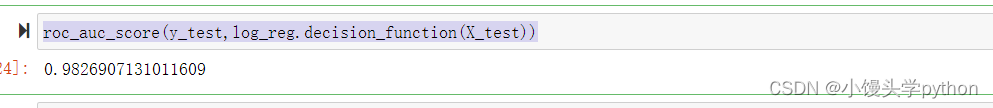### 🍋PR vs. ROC

PR曲线和ROC曲线都有其独特的用途和适用场景。PR曲线更适合于不平衡类别分布的问题，因为它关注的是正类别的准确性和召回率。ROC曲线在不同类别之间的代价不平衡问题中表现较好，因为它关注的是True Positive Rate和False Positive Rate。

## 🍋根据情境选择适当的性能度量